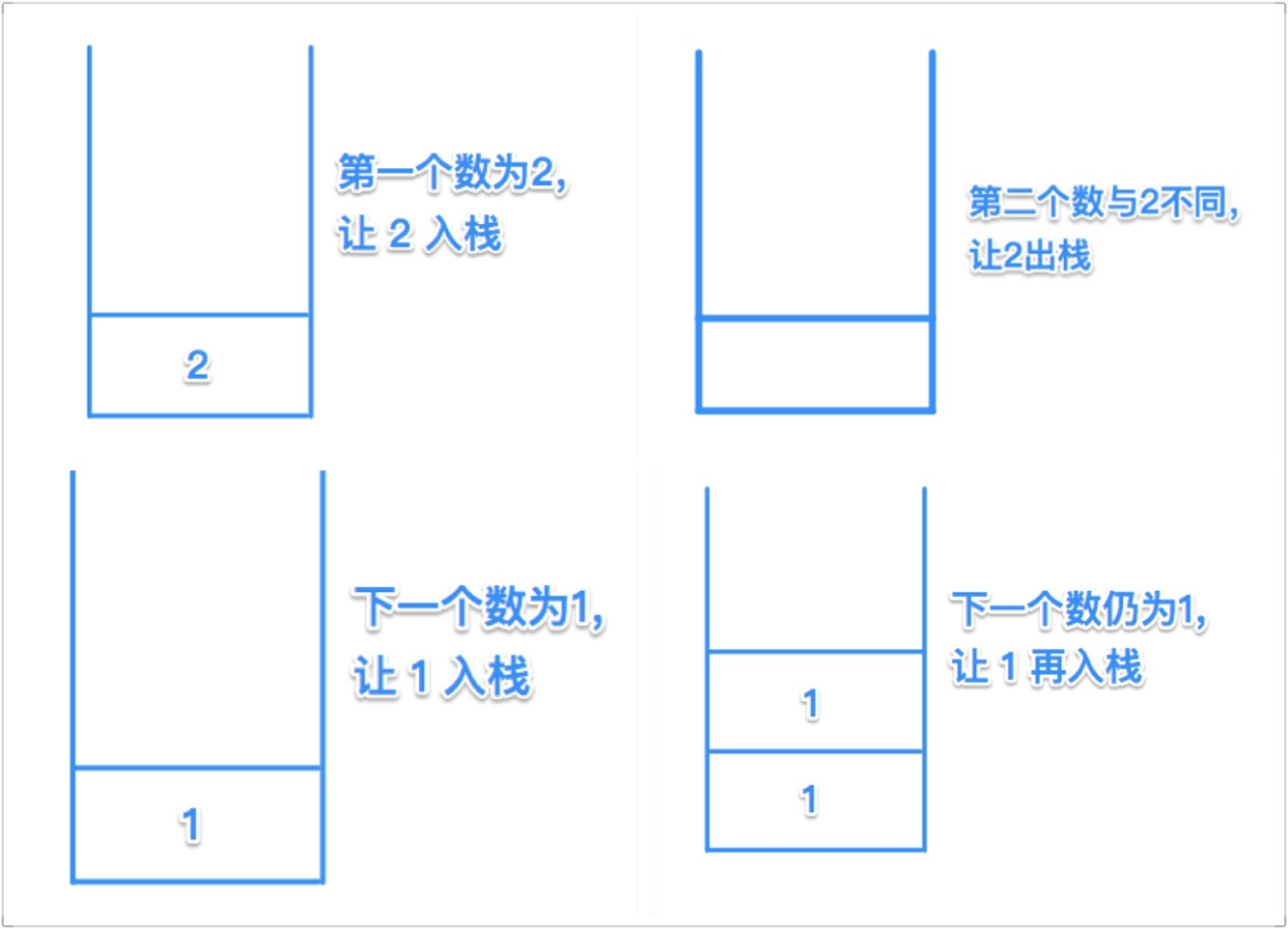# ACM：Ignatius and the Princess IV

#### Ignatius and the Princess IV

"OK, you are not too bad, em... But you can never pass the next test." feng5166 says.
"I will tell you an odd number N, and then N integers. There will be a special integer among them, you have to tell me which integer is the special one after I tell you all the integers." feng5166 says.
"But what is the characteristic of the special integer?" Ignatius asks.
"The integer will appear at least (N+1)/2 times. If you can't find the right integer, I will kill the Princess, and you will be my dinner, too. Hahahaha....." feng5166 says.
Can you find the special integer for Ignatius?

Input

The input contains several test cases. Each test case contains two lines. The first line consists of an odd integer N(1<=N<=999999) which indicate the number of the integers feng5166 will tell our hero. The second line contains the N integers. The input is terminated by the end of file.

Output

For each test case, you have to output only one line which contains the special number you have found.

Sample Input

5
1 3 2 3 3
11
1 1 1 1 1 5 5 5 5 5 5
7
1 1 1 1 1 1 1

Sample Output

3
5
1


#include <stdio.h>
#include <stdlib.h>

int a;//数较大，不用全局会栈溢出报错

int cmp(const void* a, const void* b) {
return ( *(int *)a - *(int *)b );
}//用sort时用到的函数，具体参考函数指针的知识

int main(int argc, const char * argv[]) {

int N;
while ( scanf("%d",&N) != EOF ) {
int i = 0;
for ( ; i < N; i++) {
scanf("%d", &a[i]);
}
qsort(a, N, sizeof(int), cmp);

printf("%d\n",a[N/2]);
}
return 0;
}



#include <stdio.h>

int main(int argc, const char * argv[]) {
int N;
while ( scanf("%d",&N) != EOF ) {
int a = {0};
int i = 0;
int r = 0;
for (; i < N; i++) {
int n;
scanf("%d", &n);
if (a[n] >= N/2) {
r = n;
}
a[n]++;
}
printf("%d\n", r);
}
}


2 4 1 1 2 2 3 2 2

2 2 2 2 2
4 1 1 3#include <stdio.h>
int main(int argc, const char * argv[]) {
int N;
while ( scanf("%d",&N) != EOF ) {
int spec_n = 0;//记录栈底数
int appear = 0;//记录栈中数量
int n;//记录每次手动输入的数
while (N--) {
scanf("%d", &n);
if (appear == 0) {//相当于栈空，直接入栈
appear = 1;//记录当前栈中有多少个数
spec_n = n;//记录栈底的数
}
else {//此时栈中一定有数
if (n != spec_n) {
appear--;
}
else {//此次读到的数与栈底的数相同
appear++;
}
}
}
printf("%d\n", spec_n);//最后在栈底的数
}
}